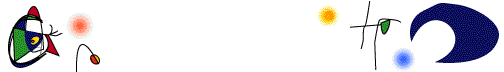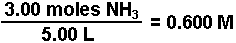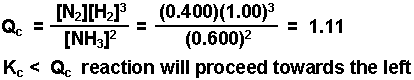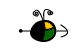Making an ICE Chart
An Aid in Solving Equilibrium Problems

An useful tool in solving equilibrium problems is an ICE chart.

• "I" stands for the initial concentrations (or pressures) for each species in the reaction mixture.
• "C" represents the change in the concentrations (or pressures) for each species as the system moves towards equilibrium.
• "E" represents the equilibrium concentrations (or pressures) of each species when the system is in a state of equilibrium.
How to Make an ICE Chart

In making an ICE chart the following items should be noted:

• Express all quantities in terms of MOLARITY (moles per liter).  (If using Kp, gaseous species must be expressed in appropriate pressure units.)
• Use initial quantities when calculating the reaction quotient, Q, to determine the direction the reaction shifts to establish equilibrium.
• Use equilibrium quantities in calculations involving the equilibrium constant, K.
• The change in each quantity must be in agreement with the reaction stoichiometry.
• Read each problem carefully to identify what quantities are given, including their unit of measure, and to identify what is unknown.
• Clearly define the change you choose to be represented by "x."  Define all other unknown changes in terms of this change.
The following is a "how to" make an ICE chart using the example to illustrate the process.

Example:  A mixture consisting initially of 3.00 moles NH3, 2.00 moles of N2, and 5.00 moles of H2, in a 5.00 L container was heated to 900 K, and allowed to reach equilibrium.  Determine the equilibrium concentration for each species present in the equilibrium mixture.

2 NH3(g)N2(g) + 3 H2(g)    Kc = 0.0076 @ 900 K

• Convert the initial quantities to molarities as shown for NH3.• Create a chart as illustrated below and enter in the known quantities.

 NH3 N2 H2 Initial Concentration (M) 0.600 0.400 1.00 Change in Conc. (M) Equilibrium Conc. (M)

• Calculate Qc and compare to Kc to determine the direction the reaction will proceed.• Assign a variable "x" that represents the change in the amount of one of the species.  The species with the lowest coefficient in the balanced equation usually is the easiest to handle when it comes to doing the math. Here let "x" = change in the amount of N2.

• Determine the change in all the other species in terms of "x."  Remember the change must be in agreement with the stoichiometry of the balanced equation, in this case 2:1:3.  Since the reaction goes in the reverse direction the concentrations of N2 and H2 gases will decrease (note the negative sign) and that of NH3 will increase.  Put these quantities into the chart (shown in red).

 NH3 N2 H2 Initial Concentration (M) 0.600 0.400 1.00 Change in Conc. (M) + 2 x - x - 3 x Equilibrium Conc. (M)

• Express the equilibrium concentrations in terms of "x" and the initial amounts (shown in green).

 NH3 N2 H2 Initial Concentration (M) 0.600 0.400 1.00 Change in Conc. (M) + 2 x - x - 3 x Equilibrium Conc. (M) 0.600 + 2 x 0.400 - x 1.00 - 3 x

• Substitute the expressions for the equilibrium concentration into the expression for the equilibrium constant and solve for "x."  Once x is known, the equilibrium concentration for each species can be calculated.The value of x was determined using the method of successive approximations.

Top

Sample Ice Charts

The following examples illustrating writing ICE charts for the problems given.  Although each problem appears to be "different" the process for creating the ICE chart is the same.

Only Reactant Species Are Present Initially

Example:  4.00 moles of HI are placed in an evacuated 5.00 L flask and then heated to 800 K.  The system is allowed to reach equilibrium.  What will be the equilibrium concentration of each species?

2 HI(g)H2(g) + I2(g)    Kc = 0.016 @ 800 K

Let "x" represent the change in concentration of the hydrogen gas.  Since we start with HI alone, the reaction must proceed to the right resulting in an increase in the amount of hydrogen gas.

 HI(g) H2(g) I2(g) Initial Concentration (M) 0.800 0 0 Change in Concentration (M) - 2 x + x + x Equilibrium Concentration (M) 0.800 - 2 x 0 + x 0 + x

Top

Only Product Species Are Present Initially

Example:  0.600 moles of NO and 0.750 moles of O2 are placed in an empty 2.00 L flask.  The system is allowed to establish equilibrium.  What will be the equilibrium concentration of each species in the flask?

2 NO2(g)2 NO(g) + O2(g)     Kc = 0.50

Let "x" represent the change in concentration of the oxygen gas.  Since only NO and O2 are present, the reaction must proceed to the left in order to establish equilibrium.  The  O2 gas will decrease in concentration over time.

 NO2(g) NO(g) O2(g) Initial Concentration (M) 0 0.300 0.375 Change in Concentration (M) + 2 x - 2 x - x Equilibirum Concentration (M) 2 x 0.300 - 2 x 0.375 - x

Top

Species Added to a System Initially in a State of Equilibrium

Example:  The concentrations of an equilibrium mixture of O2 , CO, and CO2  were 0.18 M, 0.35 M, and 0.029 M respectively.  Enough CO was added to the flask containing the equilibrium mixture to momentarily raise its concentration to 0.60 M.  What will be the concentration of each species in the flask once equilibrium has been re-established after the additional carbon monoxide was added?

2 CO2(g)2 CO(g) + O2(g)

After the addition of more CO the system is no longer in equilibrium.  The once equilibrium quantities of the other three substances are now initial quantities.  Let "x" represent the change in the amount of O2 gas.  Adding the CO will force the reaction to proceed in the reverse direction (K < Q) causing the amount of O2 to decrease.  (Note:  The equilibrium quantities given in the problem, before the addition of more CO, are also used to calculate the value of the equilibrium constant, Kc.)

 CO2(g) CO(g) O2(g) Initial Concentration (M) 0.029 0.60 0.18 Change in Concentration (M) + 2 x - 2 x - x Equilibrium Concentration (M) 0.029 + 2 x 0.60 - 2 x 0.18 - x

Top

Gaseous Species and Kp

Example:  Cl2 gas undergoes homolytic cleavage into chlorine atoms at 1100oC.  Kp at 1100oC for this process is 1.13 x 10-4.  If a sample with an initial Cl2 gas pressure of 0.500 atm was allowed to reach equilibrium, what is the total pressure in the flask?

Cl2(g)2 Cl(g)

Let "x" represent the change in the pressure of the Cl2 gas.  Since the reaction will proceed forwards to establish equilibrium the pressure of the Cl2 gas will decrease.  The total pressure at equilibrium will equal the sum of the partial pressures of each gas at equilibrium.

 Cl2(g) Cl(g) Initial Pressure (atm) 0.500 0 Change in Pressure (atm) - x + 2 x Pressure at Equilibrium (atm) 0.500 - x 0 + 2 x